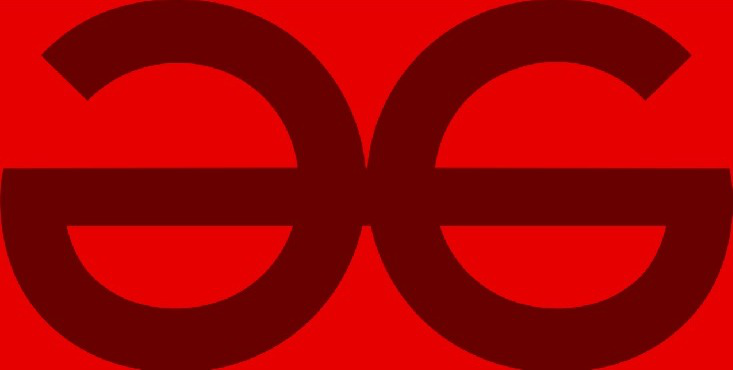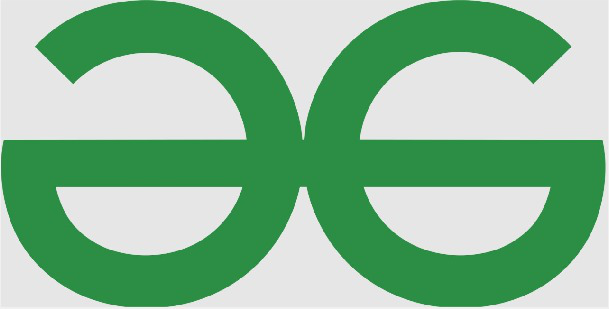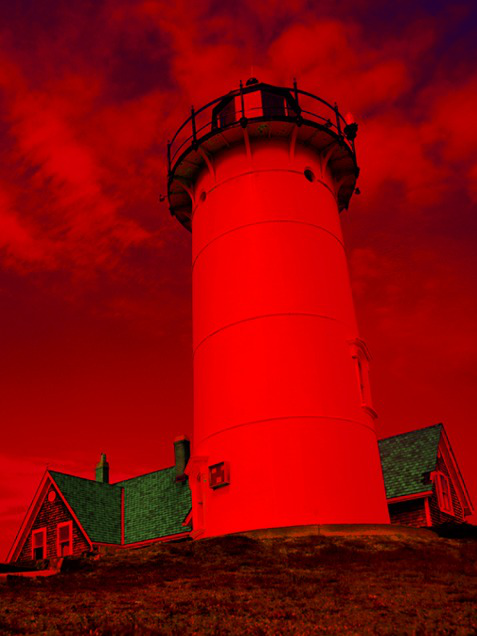# How to Convert YIQ Image to RGB Image Using MATLAB?

• Last Updated : 17 Mar, 2022

Converting images from one color space to another is a handy process in image processing that provides convenience and eases the overall process by choosing the most accurate color space to work the image on. The YIQ color space, as the name suggests comprises of three components namely Luma (Y), In-Phase(I), and Quadrature(Q).

A brief description of each component is as follows.

• Luma (Y): This describes the brightness of the image. The values lie in the range of [0,1] where 0 corresponds to black and 1 corresponds to white. The colors increase in the image as the Y value increases.
• In-Phase(I): This component gives an idea about the amount of orange and blue tones present in the image. The range of this metric is [-0.5959, 0.5959] in which the negative values correspond to the blue tones in the image and the positive values correspond to the orange tones in the image. The saturation of color is directly proportional to the I value.
• Quadrature(Q): Similar to the I component, the Q component gives a measure about the green and purple tones present in the image. The quadrature lies in the range of [-0.5229, 0.5229] where the negative values correspond to the green tones and positive values correspond to the purple tones in the image. The saturation of color is directly proportional to the magnitude of Q.
• The ntsc2rgb() function of the Image Processing Toolbox will be used to convert an image from the YIQ color space to the RGB color space.

### Approach:

• Read the input image in YIQ format using the imread() function. The imread() function in MATLAB allows the user to enable the read operation for processing.
• Use the above-mentioned function to convert the image from one color space to the other
• Display both the images together for observation purposes using the imshow() function. The imshow() function in MATLAB allows the user to enable the show/display operation for displaying the images.

We will be using the inbuilt MATLAB images for illustration purposes. If the user wishes to use any other image then one essential thing that needs to be checked is that the directory of the image and the MATLAB code are the same otherwise an error prompt would be shown. One example of this kind is also illustrated by taking the geeks for geeks logo.

The image used in the following example is a lighthouse. These images are already in RGB color space so for testing purposes we will convert to YIQ and using that as new input we will convert them back to YIQ. Default images in YIQ format are scarce in number as the color space is not commonly used.

Example 1 :

## Matlab

 `% MATLAB code for convert images in YIQ format``% Reading the Original image and``% converting to YIQ format.``% This YIQ format image will be``% effectively used as input.``% Make sure the image file path is in``% the same directory as code.``I = imread(``'GFG.jpeg'``);``J = rgb2ntsc(I);` `% Creating figure window for the input image``% Displaying the input image``figure;``imshow(J);` `% Converting YIQ to RGB``K = ntsc2rgb(J);` `% Displaying the RGB Image``figure;``imshow(K);`

Output:Figure 1: Input Image in YIQ Color SpaceFigure 2: Output Image in RGB Color Space

Example 2:

## Matlab

 `% MATLAB code``% Reading the Original image and converting``% to YIQ format. This YIQ format image will``% be effectively used as input.``I = imread(``'lighthouse.png'``);``J = rgb2ntsc(I);` `% Creating figure window for the input image``% Displaying the input image``figure;``imshow(J);` `% Converting YIQ to RGB``K = ntsc2rgb(J);` `% Displaying the RGB Image``figure;``imshow(K);`Figure 3: Input Image in YIQ color spaceFigure 4 : Output image in RGB color space

#### Code Explanation:

• I = imread(‘lighthouse.png’);  This line reads the image of the lighthouse and stores it in the variable I
• J = rgb2ntsc(I); This line converts the image to YIQ color space and assigns it to variable J ad here onwards this will be used as the input image.
• figure; This creates a window for showing an image. This particular figure window will be used to display the input image.
• imshow(J); This line of code displays the image J in the above-defined figure window.
• K = ntsc2rgb(J); This line converts the YIQ image to RGB image using the ntsc2rgb() function in MATLAB using mathematical morphological operations.
• figure; This creates a window for showing an image. This particular figure window will be used to display the output image.
• imshow(K); This line of code displays the image K in the above defined figure window. Thus we now have two figure windows side by side to simultaneously observe the differences images have when defined in different color spaces.

Thus we have achieved the task of converting an image from YIQ color space to RGB color space.

My Personal Notes arrow_drop_up